2010-07-03 15:14:00 landsuper 阅读数 6333
• ###### MATLAB图像处理

全面系统的学习MATLAB在图像处理中的应用

19908 人正在学习 去看看 魏伟

1、噪声的产生及分类:

1) 加性噪声,此类噪声与输入图象信号无关,含噪图象可表

2) 乘性噪声,此类噪声与图象信号有关,含噪图象可表示为f(x,y)=g(x,y)+n(x,y)g(x,y),飞点扫描器扫描图象时的噪声,电视图象

3) 量化噪声,此类噪声与输入图象信号无关,是量化过程存

2、去除图象噪声的方法简介:

2.1 均值滤波器

2.2 自适应维纳滤波器

2.3 中值滤波器

2.4 形态学噪声滤除器

2.5 小波去噪

2017-04-24 16:21:16 charlene_bo 阅读数 8673
• ###### MATLAB图像处理

全面系统的学习MATLAB在图像处理中的应用

19908 人正在学习 去看看 魏伟

## 1.研究噪声特性的必要性

本文的内容主要介绍了常见噪声的分类与其特性。将噪声建模，然后用模型去实现各式各样的噪声。

实际生活中的各种照片的老化，都可以归结为以下老化模型。这个模型很简单，也可以直接用以下公式来表达。根据以上式子，可以看出，老旧照片的复原，主要分为两个任务，一个是去噪；另一个是去卷积，或者称为逆滤波，也就是将老化滤波器做反处理。

本文首先由噪声类型与其建模。随后的博文，会介绍几种基础的去噪方法和基础的逆滤波方法。

## 2.噪声的实现

### 2.1    评价用图像与其直方图### 2.2  高斯噪声

高斯噪声，也称为正态噪声，其统计特性服从正态分布。一种较为泛用的噪声模型。
Matlab的实现较为简单，Matlab已经有一个randn(M,N)的函数，用其可以产生出均值为0、方差为1、尺寸为M X N像素的高斯噪声图像。
用以下程序就可以产生任意均值和方差的高斯噪声。

1. a = 0;
2. b = 0.08;
3. n_gaussian = a + b .* randn(M,N);### 2.3 瑞利噪声

瑞利噪声相比高斯噪声而言，其形状向右歪斜，这对于拟合某些歪斜直方图噪声很有用。

瑞利噪声的实现可以借由平均噪声来实现。如下所示。1. a = -0.2;
2. b = 0.03;
3. n_rayleigh = a + (-b .* log(1 - rand(M,N))).^0.5;### 2.4 伽马噪声

伽马噪声的分布，服从了伽马曲线的分布。伽马噪声的实现，需要使用b个服从指数分布的噪声叠加而来。指数分布的噪声，可以使用均匀分布来实现。1. a = 25;
2. b = 3;
3. n_Erlang = zeros(M,N);
4.
5. for j=1:b
6.     n_Erlang = n_Erlang + (-1/a)*log(1 - rand(M,N));
7. end### 2.5 均匀噪声

如同前面所示，均匀噪声可以由函数rand(M,N)直接产生。1. a = 0;
2. b = 0.3;
3. n_Uniform = a + (b-a)*rand(M,N);### 2.6 椒盐噪声

椒盐噪声也成为双脉冲噪声。在早期的印刷电影胶片上，由于胶片化学性质的不稳定和播放时候的损伤，会使得胶片表面的感光材料和胶片的基底欠落，在播放时候，产生一些或白或黑的损伤。事实上，这也可以归结为特殊的椒盐噪声。

椒盐噪声的实现，需要一些逻辑判断。这里我们的思路是，产生均匀噪声，然后将超过阈值的点设置为黑点，或白点。当然，如果需要拟合电影胶片的损伤的话，可以选用别的类型噪声去拟合。

1. a = 0.05;
2. b = 0.05;
3. x = rand(M,N);
4.
5. g_sp = zeros(M,N);
6. g_sp = f;
7.
8. g_sp(find(x<=a)) = 0;
9. g_sp(find(x > a & x<(a+b))) = 1;## 3.总结

本文，实现的几类较为基本的噪声。并给出了其实现的方法，代码在下面。下一篇博文，会进行几个常用去噪滤波器的比较。

 ``` 1 2 3 4 5 6 7 8 9 10 11 12 13 14 15 16 17 18 19 20 21 22 23 24 25 26 27 28 29 30 31 32 33 34 35 36 37 38 39 40 41 42 43 44 45 46 47 48 49 50 51 52 53 54 55 56 57 58 59 60 61 62 63 64 65 66 67 68 69 70 71 72 73 74 75 76 77 78 79 80 81 82 83 84 85 86 87 88 89 90 91 92 93 94 95 96 97 98 99 100 101 102 103 104 105 106 107 108 109 110 111 112 113 114 115 116 117 118 119 120 121 122 123 124 125 126 127 128 129 130 131 132 133 134 135 136 137 138 139 140 141 142 143 144 145 146 147 148 149 150 151 152 153 154 155 156 157 158 159 160 161 162 163 164 165 166 167 168 169 170 171 172 173``` ```close all;clear all;clc; f = imread('./original_pattern.tif');f = mat2gray(f,[0 255]);[M,N] = size(f); figure();subplot(1,2,1);imshow(f,[0 1]);xlabel('a).Original image'); subplot(1,2,2);x = linspace(-0.2,1.2,358);h = hist(f,x)/(M*N);Histogram = zeros(358,1);for y = 1:256 Histogram = Histogram + h(:,y);endbar(-0.2:1/255:1.2,Histogram);axis([-0.2 1.2 0 0.014]),grid;xlabel('b).The Histogram of a');ylabel('Number of pixels');%% ---------------gaussian-------------------a = 0;b = 0.08;n_gaussian = a + b .* randn(M,N); g_gaussian = f + n_gaussian; figure();subplot(1,2,1);imshow(g_gaussian,[0 1]);xlabel('a).Ruselt of Gaussian noise'); subplot(1,2,2);x = linspace(-0.2,1.2,358);h = hist(g_gaussian,x)/(M*N);Histogram = zeros(358,1);for y = 1:256 Histogram = Histogram + h(:,y);endbar(-0.2:1/255:1.2,Histogram);axis([-0.2 1.2 0 0.014]),grid;xlabel('b).The Histogram of a');ylabel('Number of pixels'); %% ---------------rayleigh-------------------a = -0.2;b = 0.03;n_rayleigh = a + (-b .* log(1 - rand(M,N))).^0.5; g_rayleigh = f + n_rayleigh; figure();subplot(1,2,1);imshow(g_rayleigh,[0 1]);xlabel('a).Ruselt of Rayleigh noise'); subplot(1,2,2);x = linspace(-0.2,1.2,358);h = hist(g_rayleigh,x)/(M*N);Histogram = zeros(358,1);for y = 1:256 Histogram = Histogram + h(:,y);endbar(-0.2:1/255:1.2,Histogram);axis([-0.2 1.2 0 0.014]),grid;xlabel('b).The Histogram of a');ylabel('Number of pixels');%% ---------------Erlang-------------------a = 25;b = 3;n_Erlang = zeros(M,N); for j=1:b n_Erlang = n_Erlang + (-1/a)*log(1 - rand(M,N));end g_Erlang = f + n_Erlang; figure();subplot(1,2,1);imshow(g_Erlang,[0 1]);xlabel('a).Ruselt of Erlang noise'); subplot(1,2,2);x = linspace(-0.2,1.2,358);h = hist(g_Erlang,x)/(M*N);Histogram = zeros(358,1);for y = 1:256 Histogram = Histogram + h(:,y);endbar(-0.2:1/255:1.2,Histogram);axis([-0.2 1.2 0 0.014]),grid;xlabel('b).The Histogram of a');ylabel('Number of pixels'); %% ---------------Exponential-------------------a = 9;n_Ex = (-1/a)*log(1 - rand(M,N)); g_Ex = f + n_Ex; figure();subplot(1,2,1);imshow(g_Ex,[0 1]);xlabel('a).Ruselt of Exponential noise'); subplot(1,2,2);x = linspace(-0.2,1.2,358);h = hist(g_Ex,x)/(M*N);Histogram = zeros(358,1);for y = 1:256 Histogram = Histogram + h(:,y);endbar(-0.2:1/255:1.2,Histogram);axis([-0.2 1.2 0 0.014]),grid;xlabel('b).The Histogram of a');ylabel('Number of pixels'); %% ---------------Uniform-------------------a = 0;b = 0.3;n_Uniform = a + (b-a)*rand(M,N); g_Uniform = f + n_Uniform; figure();subplot(1,2,1);imshow(g_Uniform,[0 1]);xlabel('a).Ruselt of Uniform noise'); subplot(1,2,2);x = linspace(-0.2,1.2,358);h = hist(g_Uniform,x)/(M*N);Histogram = zeros(358,1);for y = 1:256 Histogram = Histogram + h(:,y);endbar(-0.2:1/255:1.2,Histogram);axis([-0.2 1.2 0 0.014]),grid;xlabel('b).The Histogram of a');ylabel('Number of pixels'); %% ---------------Salt & pepper-------------------a = 0.05;b = 0.05;x = rand(M,N); g_sp = zeros(M,N);g_sp = f; g_sp(find(x<=a)) = 0;g_sp(find(x > a & x<(a+b))) = 1; figure();subplot(1,2,1);imshow(g_sp,[0 1]);xlabel('a).Ruselt of Salt & pepper noise'); subplot(1,2,2);x = linspace(-0.2,1.2,358);h = hist(g_sp,x)/(M*N);Histogram = zeros(358,1);for y = 1:256 Histogram = Histogram + h(:,y);endbar(-0.2:1/255:1.2,Histogram);axis([-0.2 1.2 0 0.3]),grid;xlabel('b).The Histogram of a');ylabel('Number of pixels');```
##### 来自CODE的代码片

2018-07-06 18:31:04 JonyHwang 阅读数 9699
• ###### MATLAB图像处理

全面系统的学习MATLAB在图像处理中的应用

19908 人正在学习 去看看 魏伟

>>imshow( f )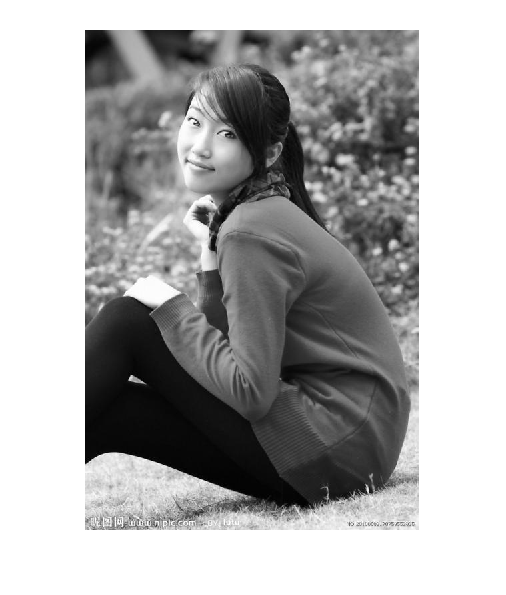>>figure,imshow( g )

>>imwrite( f, 'filename')

g=imnoise( f , type , parameters )

g=imnoise( f , 'gaussian' , m , var )将均值为m、方差为var的高斯噪声加到图像f上。默认值为均值是0、方差是0.01的噪声。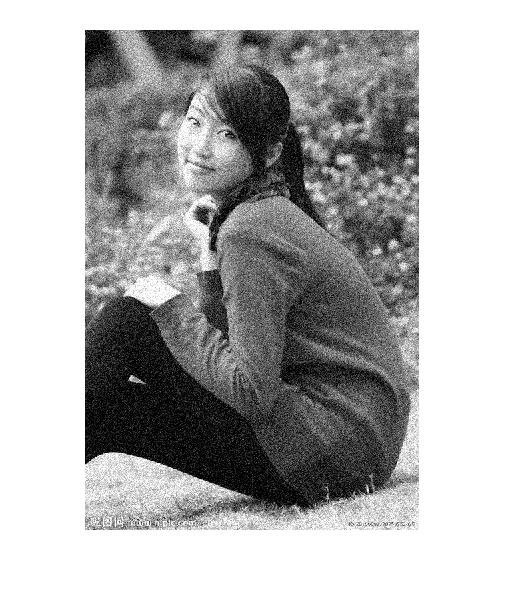g=imnoise( f , 'localvar' , V )将均值为0、局部方差为V的高斯噪声加到图像f上。其中V是与f大小相同的一个数组，它包含了每个点的理想方差值。【这个函数和下面这个函数我暂时没实现，需要找下问题，请大牛指点】

g=imnoise( f , 'localvar' , image_intensity , var )将均值为0的高斯噪声添加到图像f上，其中噪声的局部方差var是图像f的亮度值的函数。参量image_intensity和var是大小相同的向量，plot（image_intensity , var）绘制出噪声方差和图像亮度的函数关系。向量image_intensity必须包含范围在【0,1】内的归一化亮度值。

g=imnoise( f , 'salt & pepper' , d )用椒盐噪声污染图像f，其中d是噪声密度（即包含噪声值的图像区域的百分比）。因此，大约有d*numel（f）个像素收到了影响。默认噪声密度为0.05。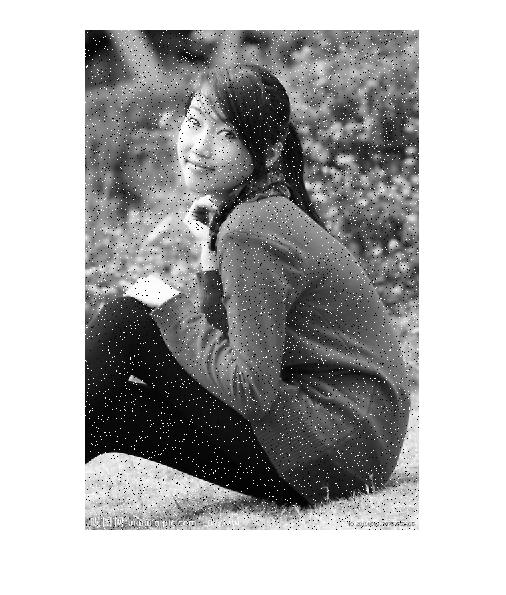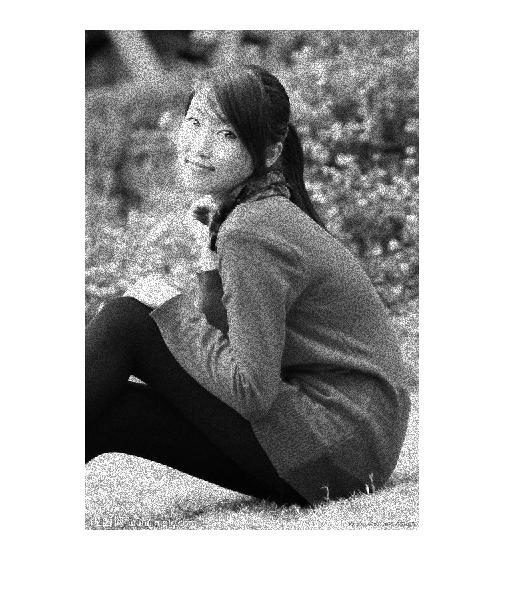g=imnoise( f , 'poisson' )从数据中生成泊松噪声。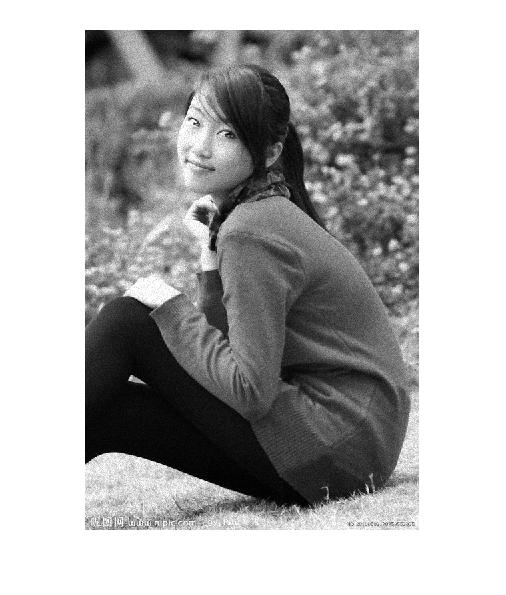2008-09-20 15:28:00 kofsky 阅读数 5946
• ###### MATLAB图像处理

全面系统的学习MATLAB在图像处理中的应用

19908 人正在学习 去看看 魏伟

傅立叶变换在图像处理中有非常非常的作用。因为不仅傅立叶分析涉及图像处理的很多方面，傅立叶的改进算法，

1.图像增强与图像去噪

2.图像分割之边缘检测

3.图像特征提取：

4.图像压缩

2015-11-11 10:32:15 cwcww1314 阅读数 1006
• ###### MATLAB图像处理

全面系统的学习MATLAB在图像处理中的应用

19908 人正在学习 去看看 魏伟

1.图像增强与图像去噪

2.图像分割之边缘检测

3.图像特征提取：

4.图像压缩

）。中值滤波器对脉冲型噪声有很好的去掉效果。因为脉冲点都是突变的点，排序以后输出中值，那么那些最大点和最小点就可以去掉了。中值滤波对高斯噪音效果较差（//高斯噪声满足高斯分布，和分布有关？）

1. 傅立叶变换在实际中有非常明显的物理意义，设f是一个能量有限的模拟信号，则其傅立叶变换就表示f的谱。从纯粹的数学意义上看，傅立叶变换是将一个函数转换为一系列周期函数来处理的。从物理效果看，傅立叶变换是将图像从空间域转换到频率域，其逆变换是将图像从频率域转换到空间域。换句话说，傅立叶变换的物理意义是将图像的灰度分布函数变换为图像的频率分布函数，傅立叶逆变换是将图像的频率分布函数变换为灰度分布函数  傅立叶变换以前，图像（未压缩的位图）是由对在连续空间（现实空间）上的采样得到一系列点的集合，我们习惯用一个二维矩阵表示空间上各点，则图像可由z=f(x,y)来表示。由于空间是三维的，图像是二维的，因此空间中物体在另一个维度上的关系就由梯度来表示，这样我们可以通过观察图像得知物体在三维空间中的对应关系。为什么要提梯度？因为实际上对图像进行二维傅立叶变换得到频谱图，就是图像梯度的分布图，当然频谱图上的各点与图像上各点并不存在一一对应的关系，即使在不移频的情况下也是没有。傅立叶频谱图上我们看到的明暗不一的亮点，实际上图像上某一点与邻域点差异的强弱，即梯度的大小，也即该点的频率的大小（可以这么理解，图像中的低频部分指低梯度的点，高频部分相反）。一般来讲，梯度大则该点的亮度强，否则该点亮度弱。这样通过观察傅立叶变换后的频谱图，也叫功率图，我们首先就可以看出，图像的能量分布，如果频谱图中暗的点数更多，那么实际图像是比较柔和的（因为各点与邻域差异都不大，梯度相对较小），反之，如果频谱图中亮的点数多，那么实际图像一定是尖锐的，边界分明且边界两边像素差异较大的。对频谱移频到原点以后，可以看出图像的频率分布是以原点为圆心，对称分布的。将频谱移频到圆心除了可以清晰地看出图像频率分布以外，还有一个好处，它可以分离出有周期性规律的干扰信号，比如正弦干扰，一副带有正弦干扰，移频到原点的频谱图上可以看出除了中心以外还存在以某一点为中心，对称分布的亮点集合，这个集合就是干扰噪音产生的，这时可以很直观的通过在该位置放置带阻滤波器消除干扰